# Progressive Quantum Leaps

### Outlining the promise of quantum computers.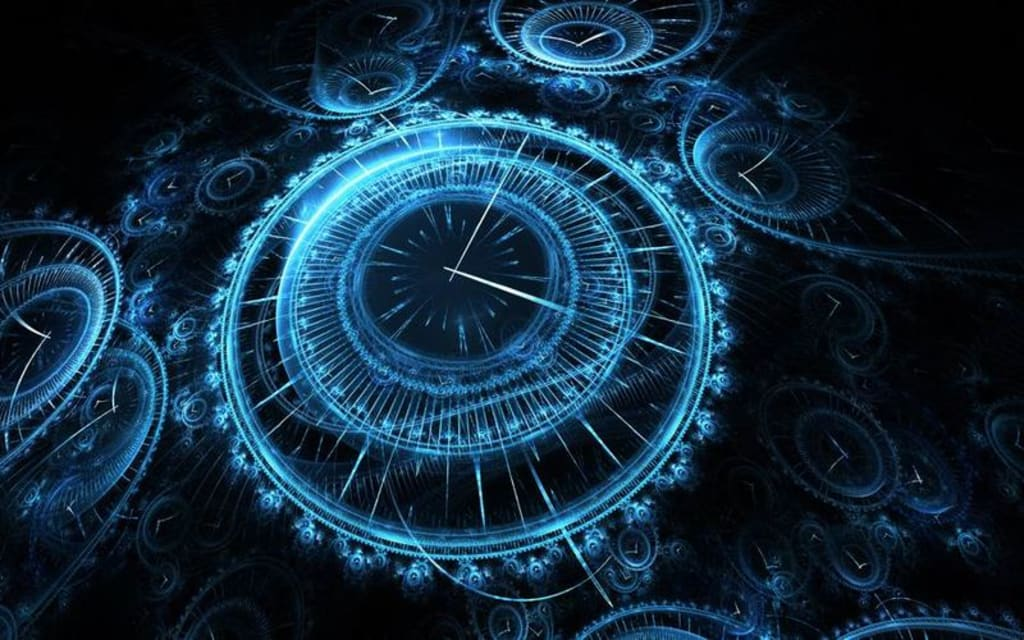In light of recent physics reports on the discovery of a new state of quantum matter formed in the lab known as ‘time crystals’ with foreseeable applications in quantum computing, the concept of quantum computing is fast becoming common parlance. It is, after all, 2017 and there is perhaps no better time for quantum leaps of awareness than right now. As a recent article published on Nature further outlines, with quantum leaps, bit by bit, quantum computers have arrived at a point where they are beginning to challenge their classical counterparts. This post is a simple, side-by-side comparison of classical computing vs. quantum computing.

### Encoding Information in Bits and Qubits

Recent progress in the interdisciplinary fields of physics, mathematics, engineering and computer science have advanced insights in quantum computing, revealing equally unique promises and challenges in the field. In classical computing, information is encoded in bits as a string of 1s or 0s (Figure 1A), so for instance 10 bits gives 2^10 or 1024 combinations of 0s and 1s, representing one number between 0 and 1023. Likewise, the quantum analogue to the classical bit of encoding information is termed qubit, but a qubit is a superimposed representation of 1s and 0s at the same time (Figure 1B), so essentially 10 qubits can simultaneously encode all 1024 numbers.

### Figure 1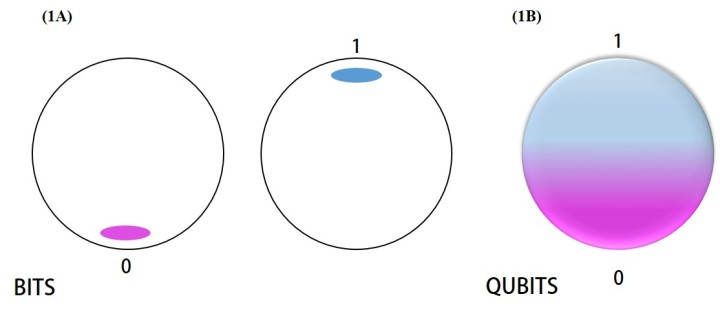1A) Bits represented as 0s and 1s in classical computing. 1B) Qubits super-imposed representing 1s and 0s at the same time in quantum computing. Figure modified from visual-science, Nature Outline.

### Operating systems in Classical and Quantum computing

Qubits can take on various physical forms, which include electrons, photons, quantum dots, trapped ions and super conducting circuits. Physicists have shown the ability to manipulate such systems with lasers or microwaves to create quantum superpositions, with the overall aim of joining many qubits together to encode a huge amount of information. Technically, when a classical computer performs operations it will operate on single bits to derive an outcome that is either 1 or 0; in contrast, a quantum computer will take the entire superposition state of qubits and transform it to another superposition state that still encodes all the numbers (Figure 2).

### Figure 2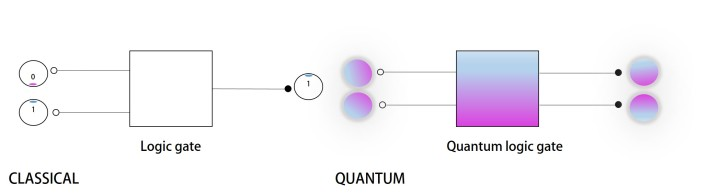A classical computer operates on single bits, deriving an outcome that is either 1 or 0. A quantum computer takes the entire superposition state of all qubits and transforms it to another superposition state that still encodes all the numbers. Figure modified from visual-science, Nature Outline.

When a qubit enters into such a state of superimposition with other qubits, such particles are said to be ‘entangled’, influencing each other in ways classical particles cannot. Additional qubits make the overall quantum state more fragile, challenging the maintenance of quantum superposition as disturbances from outside the system can destroy the coherence/consistency leading to errors or loss of quantum superposition. Whilst classical computers have established error control for robust outcomes, one option for quantum error correction is surrounding a working qubit in a quantum system by nine error-correcting qubits to restore coherence in the system known as the 9-qubit code (Figure 3, part 3).

### Figure 3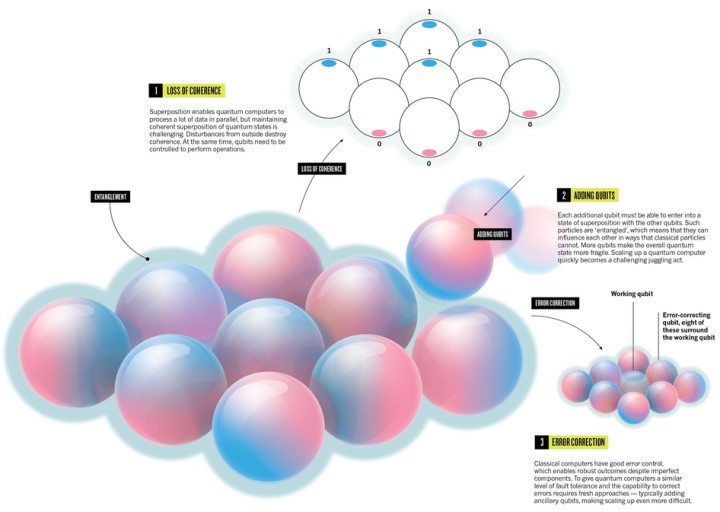Schematic representing entanglement among qubits, loss of coherence and error correction to maintain coherence. Figure obtained from visual-science, Nature Outline.

### Futuristic Game Changers

In classical computing, an algorithm is a string of operations incorporated step-by-step to solve a problem. Similarly, there are several algorithms based on quantum systems as well. Two well-known quantum algorithms, Shor’s and Grover’s algorithms, are highlighted here with their respective functions, for a realistic model of quantum computation. In general, the superposition of states in quantum systems as seen above, offer an advantage of parallelism, which means all possibilities of that system can be analysed at the same time, much like scanning a deck of cards at once to look for a specific card (Figure 4). Using Grover’s algorithm, each card will be given the probability of being ‘right’ and after several iterations, result in the cumulative probability of the target card being higher than the others. Unlike classical computing algorithms, this will be conducted individually thus consuming time. It appears that the larger the database, the bigger the advantage of incorporating quantum algorithms.

### Figure 4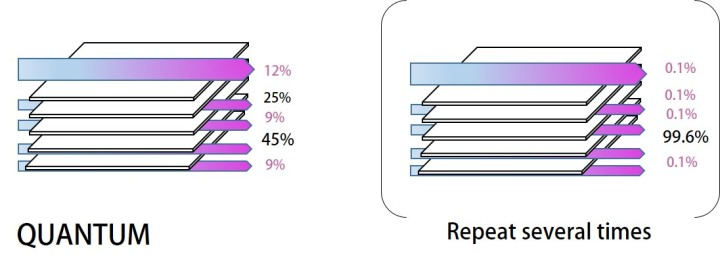Schematic visualization of using a quantum algorithm to scan through a database. Figure modified from visual-science, Nature outline.

Quantum computing will not replace classical computing but it will excel at tasks that are too complex for current computers. For instance in 1994, Peter Shor discovered that quantum computers can find prime factors of large numbers known as integer factorization, which lies at the heart of encryption (to protect online activities). For example, using Shor’s algorithm, a quantum computer may take weeks to find factors that would comparatively take classical computers thousands of years, resulting in one of the most dramatic examples of quantum “speed-up” theorized for computation, so far. Consequently, quantum states have also been implicated in creating more secure communication schemes. Furthermore, quantum computers have interdisciplinary applications, which include understanding the chemistry of molecules in relation to quantum mechanics of their electrons, and finding the optimal configuration of a folded protein as part of bioinformatics, for which a vast number of potential arrangements exist (Video 1).

So far, classical computers can calculate the simulated behavior of quantum systems of about 50 qubits. In a few years it is expected that quantum computers with more than 50 usable qubits will become available, to establish what is termed ‘quantum supremacy’. As reported in March 2017 a new quantum computing program at IBM called ‘Q’ is already planning to create just that; a quantum system with more than 50 qubits. Since qubits are known to be fragile to noise and electromagnetic radiation, the focus of the proposed 50-qubit quantum computer will be to bring stability and connectivity between qubits as well as gating and error correction, so they can be used for tasks like accelerated drug discovery and solve complex, large-scale problems with economic and scientific consequences. To recap, the implementation of quantum algorithms efficiently on a physical machine will create exciting possibilities (Video 2).

[This short article is based on the Nature|Outline report by Andreas Trabesinger, published on 22 March 2017, available at doi:10.1038/543S2a]Thamarasee Jeewandara